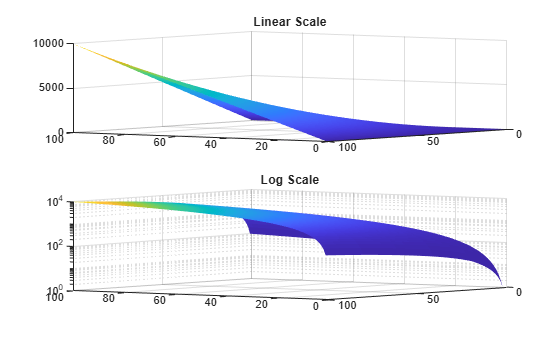# zscale

Set or query z-axis scale (linear or logarithmic)

Since R2023b

## Syntax

``zscale(scale)``
``zscale(ax,___)``
``zs = zscale``

## Description

example

````zscale(scale)` sets the scale of the z-axis to be linear or logarithmic in the current axes. Specify `scale` as `"linear"` or `"log"`. You can also omit the parentheses and quotation marks when using this syntax. For example, `zscale log` is equivalent to `zscale("log")`.```

example

````zscale(ax,___)` sets the scale for the specified axes object. This syntax is useful when you are working with multiple plots, either in a tiled chart layout or in different figures.```
````zs = zscale` returns the current z-axis scale value as `'linear'` or `'log'`.```

## Examples

collapse all

Create matrices X, Y, and Z, and use them to create a surface plot.

```[X,Y] = meshgrid(0:1:100); Z = X.*Y; surf(X,Y,Z,EdgeColor="none") view(-145,10)```Change the scale of the z-axis to logarithmic.

`zscale log`Create the same surface plot twice in a tiled chart layout. This code calls the `nexttile` function to create a tiled chart layout with one axes object. The second call to `nexttile` creates and returns the second axes object.

```[X,Y] = meshgrid(0:1:100); Z = X.*Y; nexttile; surf(X,Y,Z,EdgeColor="none") view(-145,10) title("Linear Scale") ax2 = nexttile; surf(X,Y,Z,EdgeColor="none") view(-145,10) title("Log Scale")```Change the z-axis scale of the bottom plot to logarithmic.

`zscale(ax2,"log")`## Input Arguments

collapse all

Scale value, specified as `"linear"` or `"log"`. When you change the scale, MATLAB® changes the `ZScale` property of the axes object to the value you specify.

Note

When you specify a logarithmic scale, the axes might exclude coordinates in some cases:

• If the coordinates include positive and negative values, only the positive values are displayed.

• If the coordinates are all negative, all of the values are displayed on a logarithmic scale with the appropriate sign.

• Zero values are not displayed.

Target `Axes` object. If you do not specify the target axes, `zscale` uses the current axes.

## Version History

Introduced in R2023b